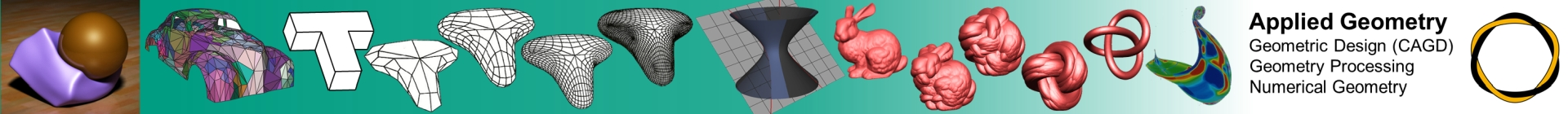### Angewandte Differentialgeometrie

This lecture supplements the lecture "Networks and Point Clouds".

In this lecture, concepts of differential geometry are discussed, which are important for computer graphics and in curves and surface design. In particular, we will discuss:

Curvatures, isophotes, geodesic lines, curvature lines, parallel curves and surfaces, minimal surfaces, low-distortion parametrizations, developable surfaces, folds.

These concepts are introduced using differentiable curves and surfaces. Based on this, the approximation and practical calculation of these concepts will be discussed. In particular, analog discrete concepts are being developed for triangular networks, which are increasingly being used for area representations.

Literature to the lecture

Datenschutz